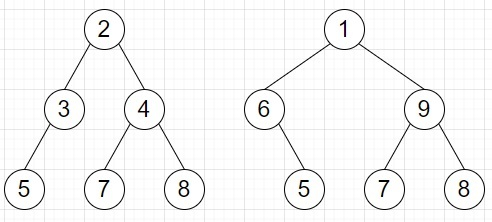# Check if leaf traversal of two Binary Trees is same in Python

Suppose we have two binary tree. We have to check whether leaf traversal of these two trees are same or not. As we know the leaf traversal is sequence of leaves traversed from left to right.

So, if the input is likethen the output will be True as the left traversal sequence of both of the trees are same, that is [5, 7, 8].

To solve this, we will follow these steps −

• s1 := a new list, s2 := another new list
• insert r1 into s1 and r2 into s2
• while s1 and s2 are not empty, do
• if s1 is empty or s2 is empty, then
• return False
• r1_node := last node of s1, and delete it from s1
• while r1_node is not same as null and r1_node is not leaf, do
• if right of r1_node is not same as null, then
• insert right of r1_node at the end of s1
• if left of r1_node is not same as null, then
• insert left of r1_node at the end of s1
• r1_node := last node of s1, and delete it from s1
• r2_node := last node of s2, and delete it from s2
• while r2_node is not null and r2_node is not leaf, do
• if right of r2_node is not null, then
• insert right of r2_node at the end of s2
• if left of r2_node is not null, then
• insert left of r2_node at the end of s2
• r2_node := last node of s2, and delete it from s2
• if r1_node is null and r2_node is not null, then
• return False
• if r1_node is not null and r2_node is null, then
• return False
• if r1_node and r2_node both are not null, then
• if value of r1_node is not same as value of r2_node, then
• return False
• return True

## Example

Let us see the following implementation to get better understanding −

Live Demo

class TreeNode:
def __init__(self, x):
self.val = x
self.left = self.right = None
def is_leaf(self):
return self.left == None and self.right == None
def solve(r1, r2):
s1 = []
s2 = []
s1.append(r1)
s2.append(r2)
while len(s1) != 0 or len(s2) != 0:
if len(s1) == 0 or len(s2) == 0:
return False
r1_node = s1.pop(-1)
while r1_node != None and not r1_node.is_leaf():
if r1_node.right != None:
s1.append(r1_node.right)
if r1_node.left != None:
s1.append(r1_node.left)
r1_node = s1.pop(-1)
r2_node = s2.pop(-1)
while r2_node != None and not r2_node.is_leaf():
if r2_node.right != None:
s2.append(r2_node.right)
if r2_node.left != None:
s2.append(r2_node.left)
r2_node = s2.pop(-1)
if r1_node == None and r2_node != None:
return False
if r1_node != None and r2_node == None:
return False
if r1_node != None and r2_node != None:
if r1_node.val != r2_node.val:
return False
return True
root1 = TreeNode(2)
root1.left = TreeNode(3)
root1.right = TreeNode(4)
root1.left.left = TreeNode(5)
root1.right.left = TreeNode(7)
root1.right.right = TreeNode(8)
root2 = TreeNode(1)
root2.left = TreeNode(6)
root2.right = TreeNode(9)
root2.left.right = TreeNode(5)
root2.right.left = TreeNode(7)
root2.right.right = TreeNode(8)
print(solve(root1, root2))

## Input

root1 = TreeNode(2)
root1.left = TreeNode(3)
root1.right = TreeNode(4)
root1.left.left = TreeNode(5)
root1.right.left = TreeNode(7)
root1.right.right = TreeNode(8)
root2 = TreeNode(1)
root2.left = TreeNode(6)
root2.right = TreeNode(9)
root2.left.right = TreeNode(5)
root2.right.left = TreeNode(7)
root2.right.right = TreeNode(8)

## Output

True